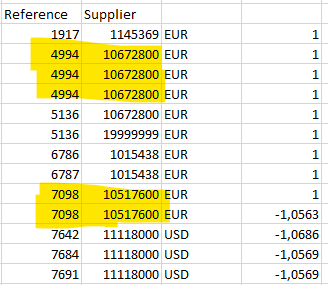# Excel read out and filter by two columns

Hi,

I would like to have all transactions where the value from the Reference and Supplier columns are identical.

exampl.:I need a DataTable only with this referencesTanks
Nina

@nina.wenner

`dt = dt.AsEnumerable.GroupBy(function(x) x("Reference").ToString+x("Supplier").ToSTring).Where(function(x) x.Count>1).SelectMany(function(x) x).CopyToDataTable`

cheers

@nina.wenner

``````dt1.AsEnumerable.GroupBy(function(a) Tuple.Create(a(0).tostring,a(1).ToString)).where(Function(grp) grp.Count>1).SelectMany(function(grp) grp).copytodatatable
``````

you can also try in this way

Assign activity
dtResult | DataTable =

``````(From d in YourOrigDataVar.asEnumerable
Group d by k1=d("Reference").toString.Trim, k2=d("Supplier").toString.Trim into grp=Group
Where grp.Count > 1
From g in grp
Select r=g).CopyToDataTable
``````

@Anil_G thank you very much it’s working.

1 Like

This topic was automatically closed 3 days after the last reply. New replies are no longer allowed.